# Question Question Question Question 2 An electric field

• Slides: 30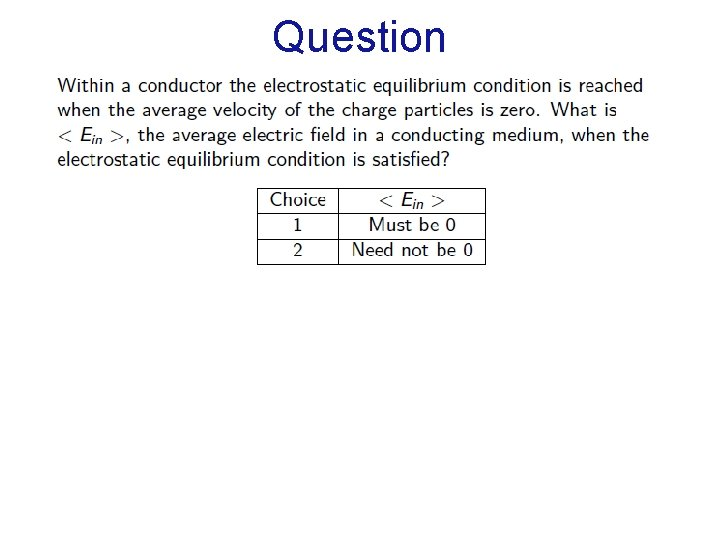Question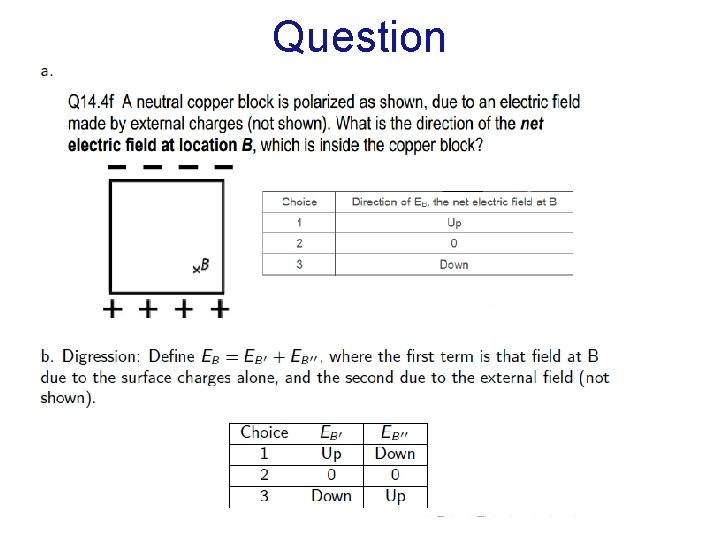QuestionQuestion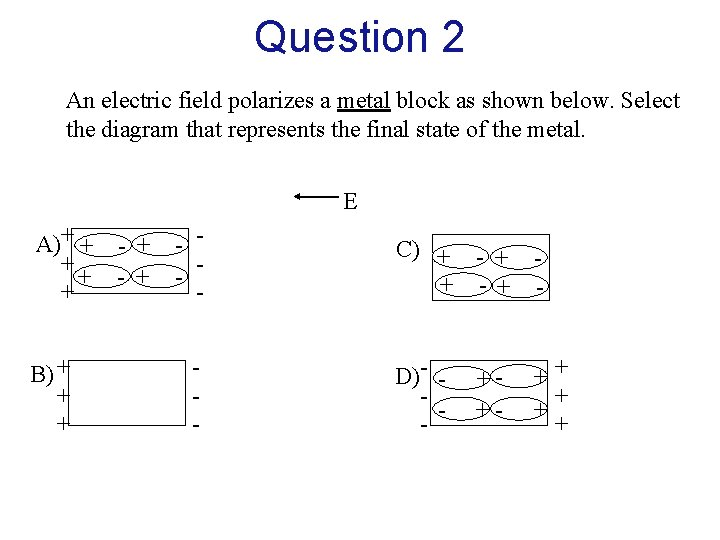Question 2 An electric field polarizes a metal block as shown below. Select the diagram that represents the final state of the metal. E A)+ + - + + B) + + + - - C) + - + D)- - ++ + + +Chapter 16 Electric Field of Distributed ChargesDistributed ChargesUniformly Charged Thin Rod Length: L Charge: Q What is the pattern of electric field around the rod? Cylindrical symmetry Could the rod be a conductor and be uniformly charged?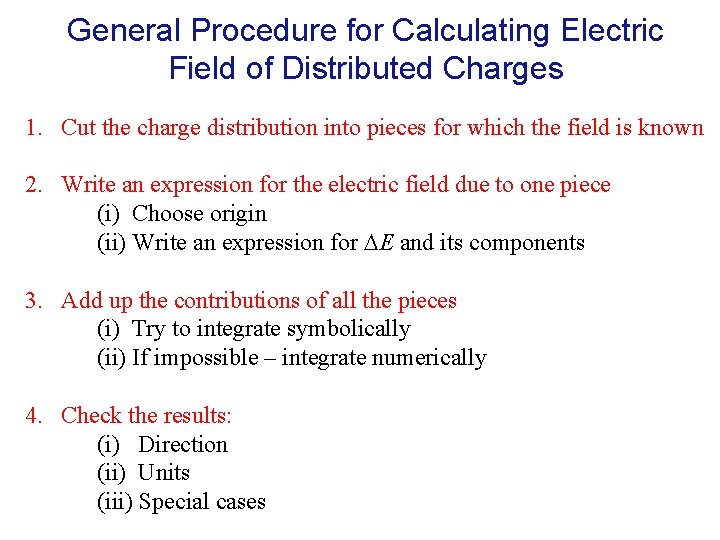General Procedure for Calculating Electric Field of Distributed Charges 1. Cut the charge distribution into pieces for which the field is known 2. Write an expression for the electric field due to one piece (i) Choose origin (ii) Write an expression for E and its components 3. Add up the contributions of all the pieces (i) Try to integrate symbolically (ii) If impossible – integrate numerically 4. Check the results: (i) Direction (ii) Units (iii) Special cases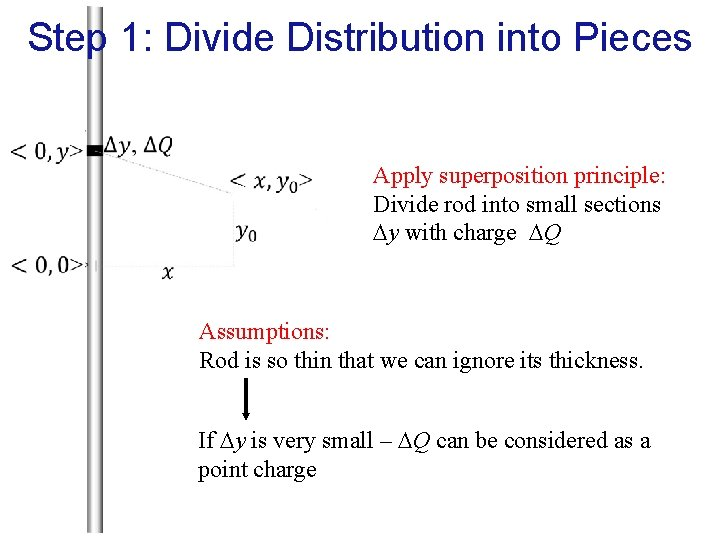Step 1: Divide Distribution into Pieces Apply superposition principle: Divide rod into small sections y with charge Q Assumptions: Rod is so thin that we can ignore its thickness. If y is very small – Q can be considered as a point charge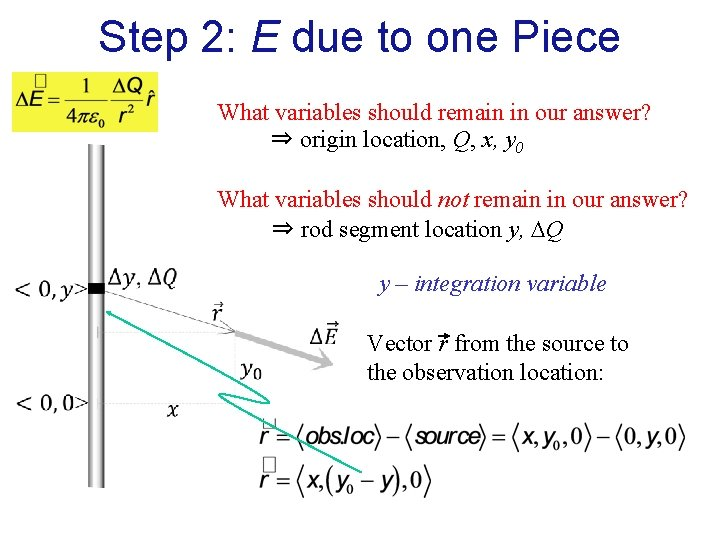Step 2: E due to one Piece What variables should remain in our answer? ⇒ origin location, Q, x, y 0 What variables should not remain in our answer? ⇒ rod segment location y, Q y – integration variable Vector r from the source to the observation location: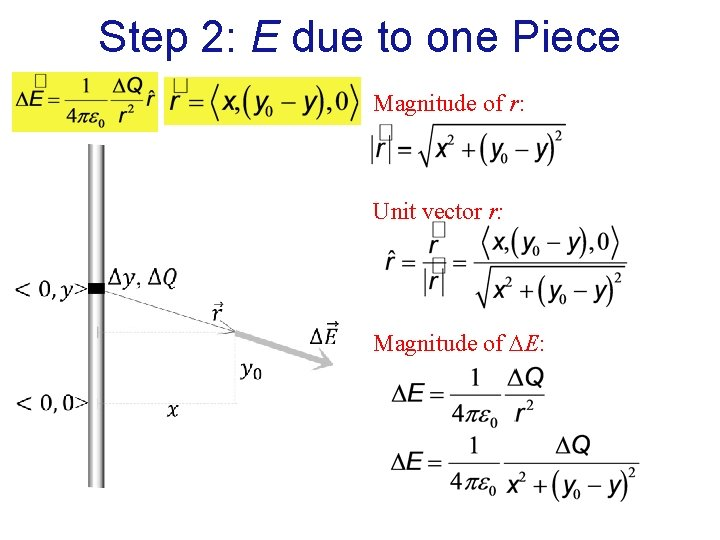Step 2: E due to one Piece Magnitude of r: Unit vector r: Magnitude of E:Step 2: E due to one Piece Vector ΔE: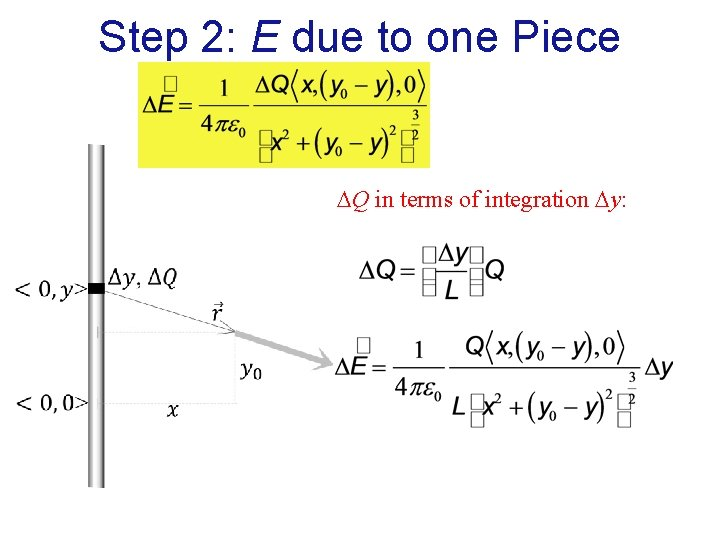Step 2: E due to one Piece Q in terms of integration y: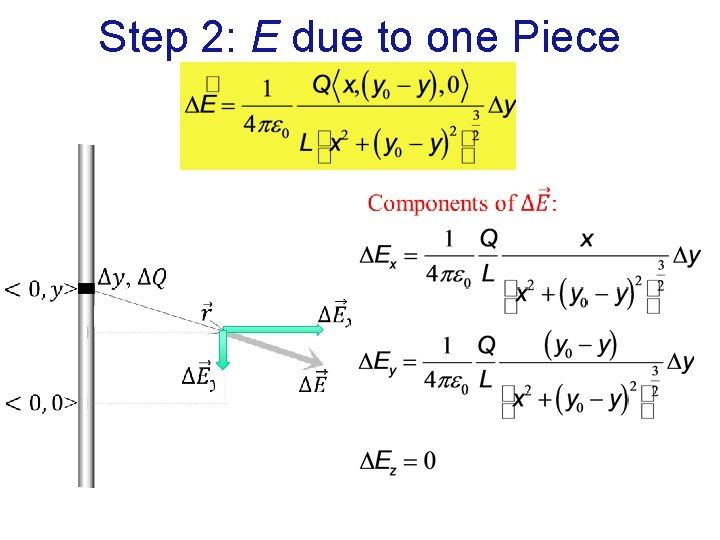Step 2: E due to one Piece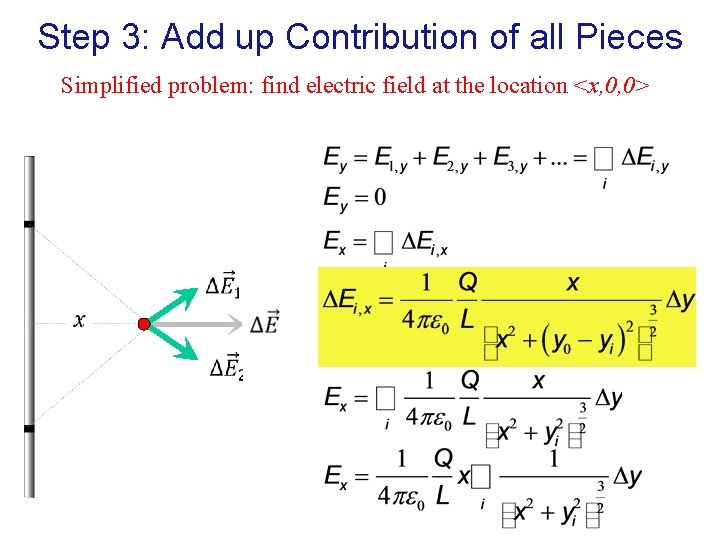Step 3: Add up Contribution of all Pieces Simplified problem: find electric field at the location <x, 0, 0>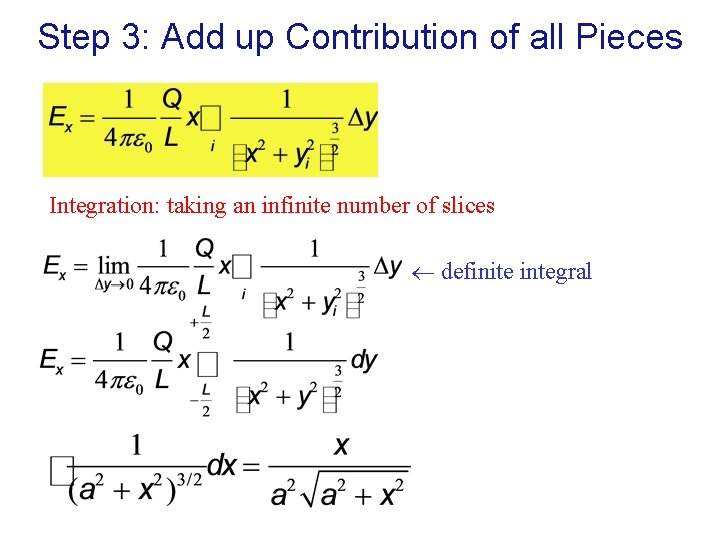Step 3: Add up Contribution of all Pieces Integration: taking an infinite number of slices definite integral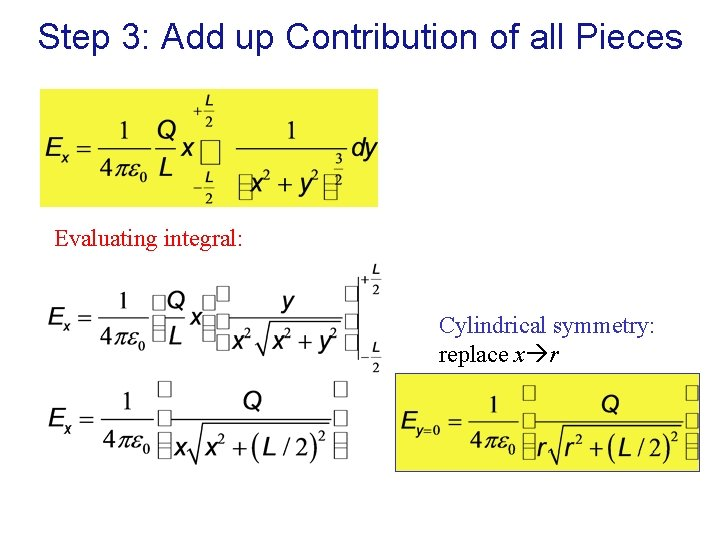Step 3: Add up Contribution of all Pieces Evaluating integral: Cylindrical symmetry: replace x rE of Uniformly Charged Thin Rod At center plane In vector form: Step 4: Check the results: Direction: Units: Special case r>>L: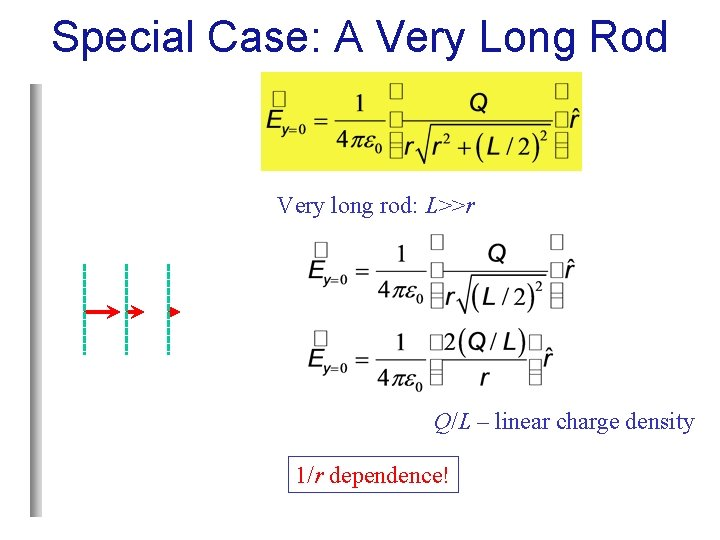Special Case: A Very Long Rod Very long rod: L>>r Q/L – linear charge density 1/r dependence!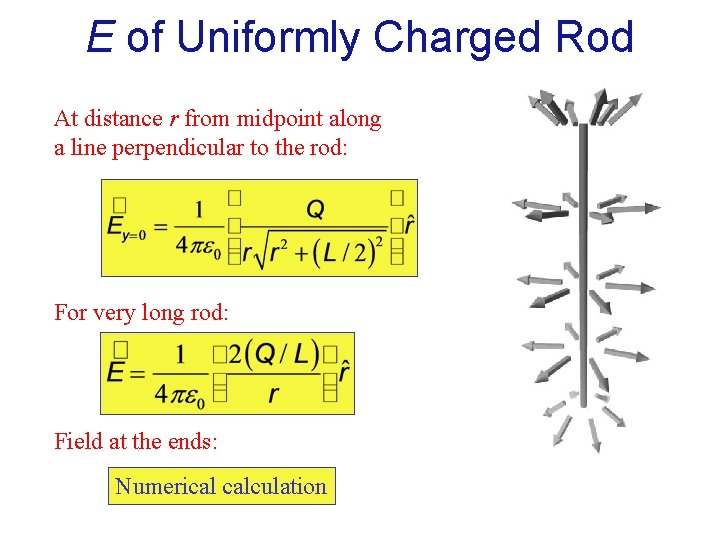E of Uniformly Charged Rod At distance r from midpoint along a line perpendicular to the rod: For very long rod: Field at the ends: Numerical calculationGeneral Procedure for Calculating Electric Field of Distributed Charges 1. Cut the charge distribution into pieces for which the field is known 2. Write an expression for the electric field due to one piece (i) Choose origin (ii) Write an expression for E and its components 3. Add up the contributions of all the pieces (i) Try to integrate symbolically (ii) If impossible – integrate numerically 4. Check the results: (i) Direction (ii) Units (iii) Special cases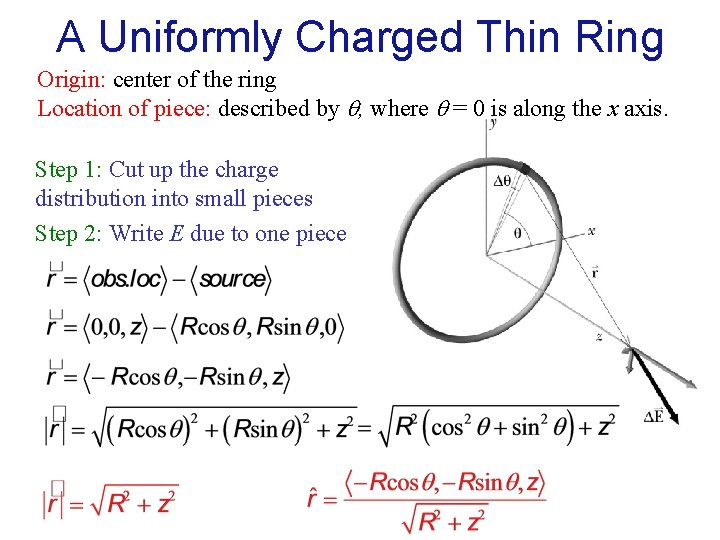A Uniformly Charged Thin Ring Origin: center of the ring Location of piece: described by q, where q = 0 is along the x axis. Step 1: Cut up the charge distribution into small pieces Step 2: Write E due to one piece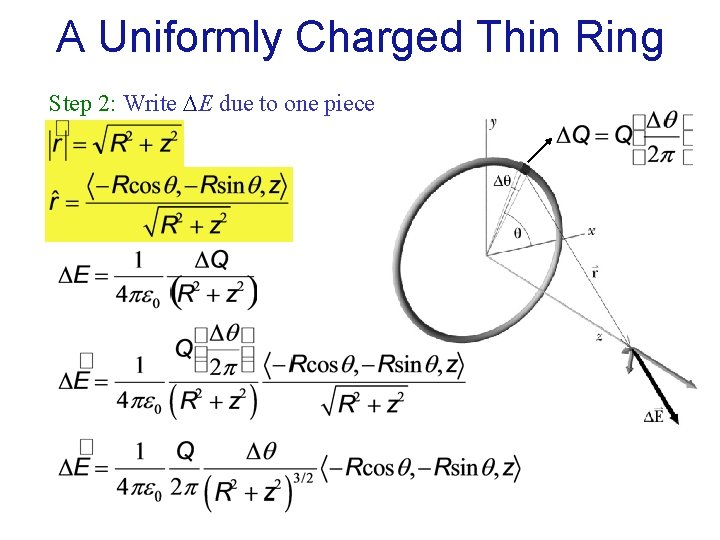A Uniformly Charged Thin Ring Step 2: Write E due to one piece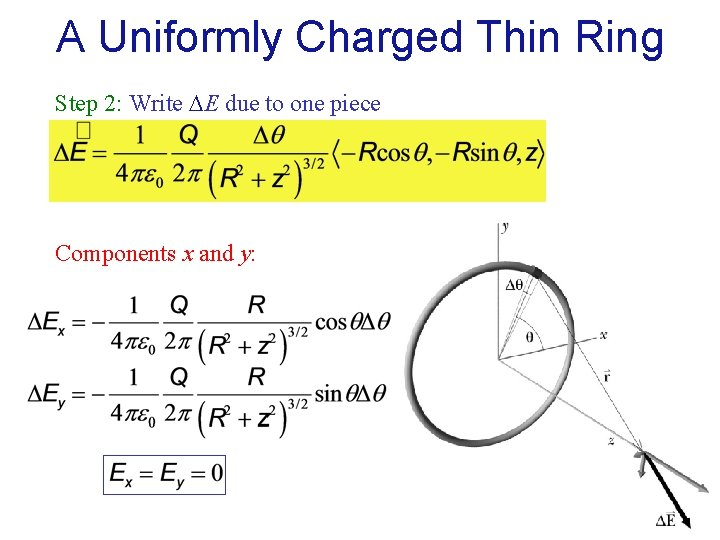A Uniformly Charged Thin Ring Step 2: Write E due to one piece Components x and y: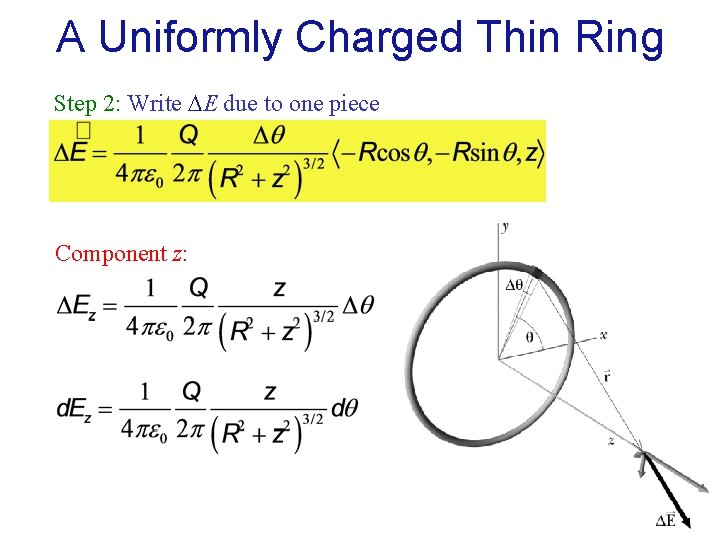A Uniformly Charged Thin Ring Step 2: Write E due to one piece Component z:A Uniformly Charged Thin Ring Step 3: Add up the contributions of all the piecesA Uniformly Charged Thin Ring Step 4: Check the results Direction Units Special cases: Center of the ring (z=0): Ez=0 Far from the ring (z>>R):A Uniformly Charged Thin Ring Distance dependence: Far from the ring (z>>R): Ez~1/z 2 Close to the ring (z<<R): Ez~z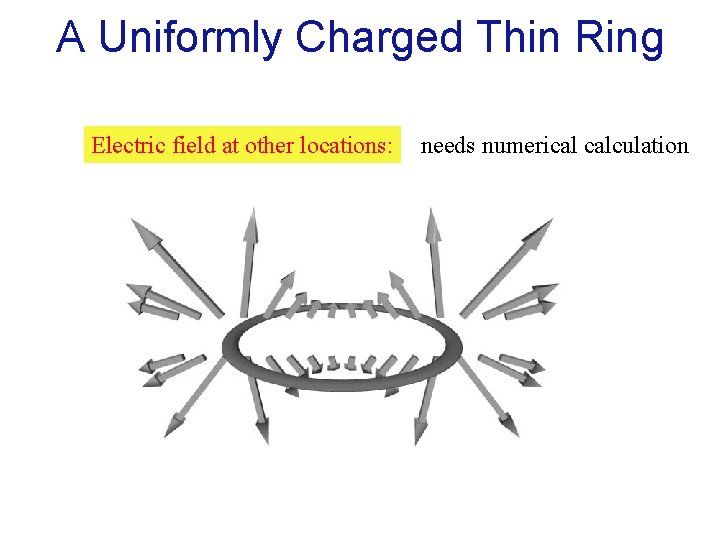A Uniformly Charged Thin Ring Electric field at other locations: needs numerical calculationA Uniformly Charged Disk Section 16. 5 – Study this!